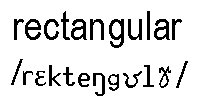IN MATH: 1. a quadrilateral with 4 right angles and two sets of congruent sides. EX. A square is also a rectangle because it has 4 right angles and all congruent sides, not just two sets of congruent sides.

 See:WALK AROUND WITH ME - perimeter, polygons VOCABULARY

IN ENGLISH: 1. as defined above.

APPLICATION: See list 140.

1. A rectangle has a width of 4 m and a length of 6 meters. Find its perimeter and area.

2. A rectangle has a width of x meters and a length of 3x + 4 meters. Find its perimeter and area.IN MATH: 1. adj. having the shape of a rectangle. 2. adj. a graphing display using the Cartesian coordinate system rather than POLAR coordinates.

APPLICATION: See list 140 and 400.This is a page from the dictionary MATH SPOKEN HERE!, published in 1995 by MATHEMATICAL CONCEPTS, inc., ISBN: 0-9623593-5-1.   You are hereby granted permission to make ONE printed copy of this page and its picture(s) for your PERSONAL and not-for-profit use.